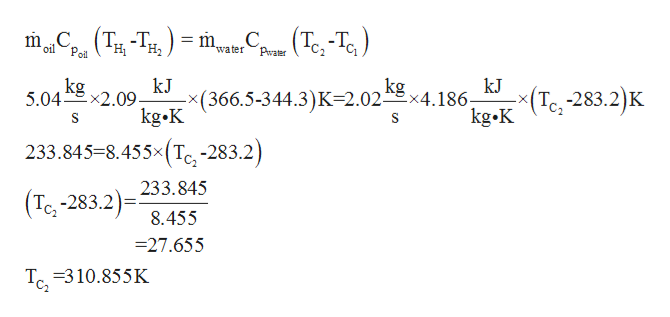# Cooling Oil by Water in an Exchanger. Oil flowing at the rate of 5.04 kg/s (cpm2.09 kJ/kg K) is cooled in a 1-2 heat exchanger from 366.5 K to 344.3 K by 2.02 kg/sof water entering at 283.2 K.The overall heat-transfer coefficient U, is 340 W/m2. K.Calculate the area required. (Hint: A heat balance must first be made to determinethe outlet water temperature.)

Question
147 viewshelp_outlineImage TranscriptioncloseCooling Oil by Water in an Exchanger. Oil flowing at the rate of 5.04 kg/s (cpm 2.09 kJ/kg K) is cooled in a 1-2 heat exchanger from 366.5 K to 344.3 K by 2.02 kg/s of water entering at 283.2 K.The overall heat-transfer coefficient U, is 340 W/m2. K. Calculate the area required. (Hint: A heat balance must first be made to determine the outlet water temperature.) fullscreen
check_circle

Step 1

Heat exchanger is a mechanical device which is used to transfer heat between two fluids. One fluid is heated or cooled with the help of another fluid. Given heat exchanger is 1-2(one shell and two tubes) heat exchanger. These numbers show number of shells and number of tubes respectively.

Step 2

Applying energy balance on heat exchanger to find out outlet temperature of water

Amount of heat lose by hot fluid = amount of heat gained by cold fluid

Where TH1 = Inlet temperature of hot (oil) fluid

TH2 =Outlet temperature of hot (oil) fluid

TC1 = Inlet temperature of cold (water) fluid

TC2= Outlet temperature of cold (water) fluid

m∙oil = mass flow rate of oil

m∙water = mass flow rate of water

Cp(oil) =specific heat of oil

Cp(water) =specific heat of water

= 4.186 kJ/kg ∙ K

Step 3

Plugging the ...help_outlineImage Transcriptionclose,(TH-TH) .(Tc,-Te) Т. -Т. Pol С. Pwater m, oil water kg 5.04 x2.09 kg kJ -x(366.5-344.3)K-2.02x4.186 kg.K kJ -x(T -283.2)K S ) 233.845-8.455x(T -283.2 233.845 (Tc -283.2 8.455 =27.655 Te310.855K fullscreen

### Want to see the full answer?

See Solution

#### Want to see this answer and more?

Solutions are written by subject experts who are available 24/7. Questions are typically answered within 1 hour.*

See Solution
*Response times may vary by subject and question.
Tagged in

### Heat Transfer